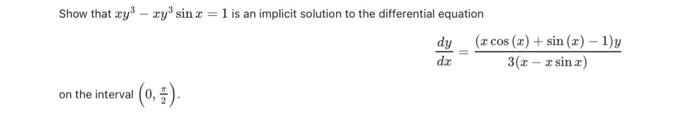Home / Expert Answers / Calculus / show-that-x-y-3-x-y-3-sin-x-1-is-an-implicit-solution-to-the-differential-equation-f-pa989

# (Solved): Show that $$x y^{3}-x y^{3} \sin x=1$$ is an implicit solution to the differential equation $\f ...Show that $$x y^{3}-x y^{3} \sin x=1$$ is an implicit solution to the differential equation \[ \frac{d y}{d x}=\frac{(x \cos (x)+\sin (x)-1) y}{3(x-x \sin x)}$ on the interval $$\left(0, \frac{\pi}{2}\right)$$.

We have an Answer from Expert